Introduction

Forward Error Correction (FEC) codes can detect and correct a limited number of errors without retransmitting the data stream. There are two basic types of FEC codes: Block codes and Convolution codes.

The significant example of Block code is BCH code. As other block code, BCH encodes k data bits into n code bits by adding n-k parity checking bits for the purpose of detecting and checking the errors.  Given the length of the codes is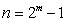for any integer mˇÝ3, we will have t (where t<), is the bound of the error correction. That is, BCH can correct any combination of errors (burst or separate) fewer than t in the n-bit-codes. The number of parity checking bits is n-kˇÜmt.

An important concept for BCH is Galois Fields (GF), which is a finite set of elements on which two binary addition and multiplication can be defined. For any prime number p there is GF(p) and GF(is called extended field of GF(p). We often use GF(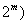in BCH code. A GF can be constructed over a primitive polynomial such as(The construction and arithmetic of GF are in ˇ°Error Control Codingˇ±, by Shu Lin). Usually, GF table records all the variables, including expressions for the elements, minimal polynomial, and generator polynomial. By referring to the table, we can locate a proper generator polynomial for encoder. For example, when (n, k, t)=(15, 7, 2), a possible generator is. If we have a data stream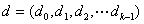, the codeword would beand have the style of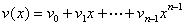.

The decoder of BCH is complicated because it has to locate and correct the errors. Suppose we have a received codeword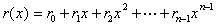, then, where, v(x) is correct codeword and e(x) is the error. First, we must compute a syndrome vector s=, which can be achieved by calculating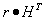, where, H is parity-check matrix and can be defined as:.

Here,is the element of the GF field and can be located in the GF table.

With syndrome, error-location polynomialcan be determined. BerkekampˇŻs iterative algorithm is one of solutions to calculate the error-location polynomial. By finding roots of, the location numbers for the errors will be achieved.

Usage

The program is developed with Java applet. Basically, the implementation involves three steps: Encoder, Error adding, Decoder.

ˇ¤      Encoder

m and t are available for adjusting. As mentioned above, the codeword length will be. t is the bound of error correction. With m and t being settled, the length of data bits is k=n-mt.  Although the program itself has no boundary for m, considering the display limitation, m will be set between 3 and 7. The range checking for m and t are available, if m and t are set to unreasonable values, a red color will be filled input area and program will keep wait for proper inputs.

. User will be able to choose either to manually type data stream in binary (user generate) or allow program to randomly generate data stream (randomly generate). If ˇ°user generateˇ± is chosen, user has to type the data stream, whose length is exact K=n-mt. Otherwise, when ˇ°user genˇ± being click, a range checking function will fill the input area with red, indicating the data length is incorrect. If ˇ°randomly generateˇ± is selected, upon m and t being settled, the program will randomly generate a data stream and encoder it into codeword.
With the ˇ°random genˇ± or ˇ°user genˇ± button being clicked, the original signal, BCH codeword and its generator sequence (, It comes from GF and constructs the codeword) will be displayed. Meanwhile, the data stream and BCH codeword will be show the plot graph underneath.

In order to evaluate the capability of BCH codes, user is allowed to decide number of errors that will happen to codeword. Besides, two error-assignment styles will be available to be selected:

Random: The faulty bits will be randomly selected from the codeword;

Burst:  The faulty bits will be consecutive, and user is allowed to set start position for burst error. In random styles, the start position will be set to ˇ°-1ˇ±, meaning not available for adjustment.  If the start position that user set exceeds the length of the codeword, the program will coerce the start position back to signal range. It is well know that burst errors are hard for normal other error correction codes to deal with. But user will find burst or separate errors make no difference for BCH code, which is an advantage of BCH code. When ˇ°adding errorˇ± is clicked, the receiving code with error bits will be displayed. Meanwhile, corresponding receiving code will be shown in the plot graph. On the receiving curve, a red ˇ°Eˇ± will appear on the error bit.

ˇ¤      Decoder

The decoder will be triggered by user clicking the ˇ°decodeˇ±. The decoder will give the results of error corrections, including:

ˇ¤       decoded signal;

ˇ¤       sigma(x):, it is the error-location polynomial and calculated by BerkekampˇŻs iterative algorithm, which is a quite complex procedure;

ˇ¤       root: the roots forand they are the position number of errors;

ˇ¤       S(x): Syndrome, which is essential for the decoder (details are discussed above);

ˇ¤       The number of corrected errors;

ˇ¤       The text message, which indicates whether all the error bits have been corrected.

The decoded signal will also be shown in the plot graph. If the number of errors exceeds t, the decoder will fail to correct all the errors. In that case, some of ˇ°Eˇ±s will be left to indicate that some errors are still there.

Reference:

 Shu Lin, ˇ°Error Control Coding: Fundamentals and Applicationsˇ±, Englewood Cliffs, N.J., Prentice-Hall, 1983.

 William Stallings, ˇ°Wireless Communications and Networksˇ±, Prentice Hall, 2002.